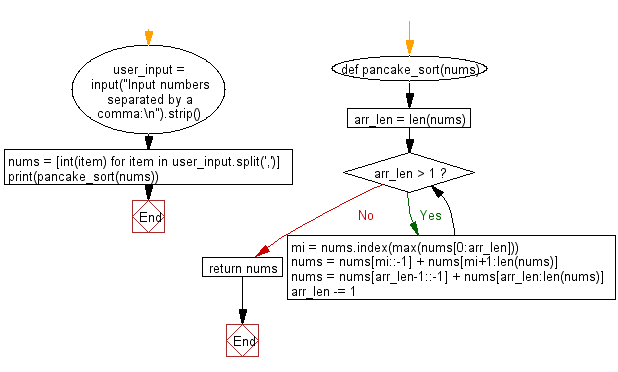﻿ Python: Sort a list of elements using Pancake sort - w3resource# Python: Sort a list of elements using Pancake sort

## Python Search and Sorting : Exercise-18 with Solution

Write a Python program to sort a list of elements using Pancake sort.
Pancake sorting is the colloquial term for the mathematical problem of sorting a disordered stack of pancakes in order of size when a spatula can be inserted at any point in the stack and used to flip all pancakes above it. A pancake number is the minimum number of flips required for a given number of pancakes. The problem was first discussed by American geometer Jacob E. Goodman. It is a variation of the sorting problem in which the only allowed operation is to reverse the elements of some prefix of the sequence.

Sample Solution:

Python Code:

``````def pancake_sort(nums):
arr_len = len(nums)
while arr_len > 1:
mi = nums.index(max(nums[0:arr_len]))
nums = nums[mi::-1] + nums[mi+1:len(nums)]
nums = nums[arr_len-1::-1] + nums[arr_len:len(nums)]
arr_len -= 1
return nums

user_input = input("Input numbers separated by a comma:\n").strip()
nums = [int(item) for item in user_input.split(',')]
print(pancake_sort(nums))
```
```

Sample Output:

```Input numbers separated by a comma:
15, 79, 25, 38, 69
[15, 25, 38, 69, 79]
```

Flowchart:Python Code Editor:

What is the difficulty level of this exercise?

Test your Programming skills with w3resource's quiz.

﻿

## Python: Tips of the Day

The Zip() Function:

```>>> students = ('John', 'Mary', 'Mike')
>>> ages = (15, 17, 16)
>>> scores = (90, 88, 82, 17, 14)
>>> for student, age, score in zip(students, ages, scores):
...     print(f'{student}, age: {age}, score: {score}')
...
John, age: 15, score: 90
Mary, age: 17, score: 88
Mike, age: 16, score: 82
>>> zipped = zip(students, ages, scores)
>>> a, b, c = zip(*zipped)
>>> print(b)
(15, 17, 16)
```